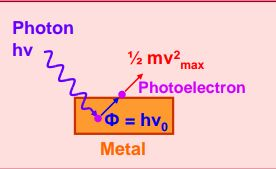Q

# Help me understand! - Dual Nature of Matter and Radiation - JEE Main

According to Einstein’s photoelectric equation, the plot of the kinetic energy of the emitted photo electrons from a metal the frequency, of the incident radiation gives a straight line whose slope

 Option 1) depends on the nature of the metal used Option 2) depends on the intensity of the radiation Option 3) depends both on the intensity of the radiation and the metal used Option 4) is the same for all metals and independent of the intensity of the radiation.
137 Views

As we learnt in

Conservation of energy -

- whereinSlope of this graph is h (planck constant). It is independent of nature of metal and intensity of light.

Correct option is 4

Option 1)

depends on the nature of the metal used

This is an incorrect option.

Option 2)

depends on the intensity of the radiation

This is an incorrect option.

Option 3)

depends both on the intensity of the radiation and the metal used

This is an incorrect option.

Option 4)

is the same for all metals and independent of the intensity of the radiation.

This is the correct option.

Exams
Articles
Questions